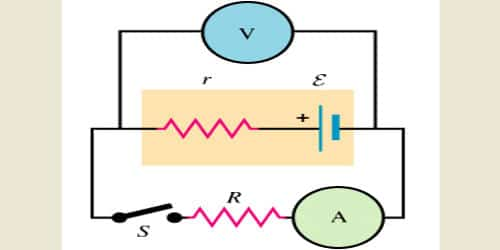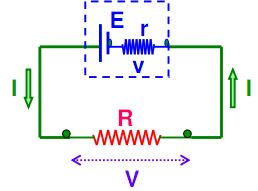Physics

# What is Internal Resistance of a Cell?Internal resistance typically means the electrical resistance inside batteries and power supplies that can limit the potential difference that can be supplied to an external load. The electric current in an external circuit flows from the positive terminal to the negative terminal of the cell, through different circuit elements. In order to maintain continuity, the current has to flow through the electrolyte of the cell, from its negative terminal to the positive terminal. During this process of flow of current inside the cell, resistance is offered to current flow by the electrolyte of the cell. This is termed as the internal resistance of the cell.

Consider the circuit given below. The cell can be modified with an emf ‘E’ and the internal resistor with resistance r which is connected in series. An external load resistor with resistance R is also connected across the circuit. The terminal potential difference represented as V is defined as the potential difference between the positive and negative terminals of the cell when current flows through the circuit.For example, a battery might have an EMF (Electromotive force – badly named term for the amount of potential difference that could be supplied under ideal conditions) of 1.60 volts, but not all of this will end up across an external load.

Internal resistance refers to the opposition to the flow of currently offered by the cells and batteries themselves resulting in the generation of heat. Internal resistance is measured in Ohms. The relationship between internal resistance (r) and emf (e) of cell s given by.

e = I (r + R)

Where, e = EMF i.e. electromotive force (Volts), I = current (A), R = Load resistance, and r is the internal resistance of cell measured in ohms.

On rearranging the above equation we get;

e = IR + Ir  or, e = V + Ir

In the above equation, V is the potential difference (terminal) across the cell when the current (I) is flowing through the circuit.

Note: The emf (e) of a cell is always greater than the potential difference (terminal) across the cell.

Example: The potential difference across the cell when no current flows through the circuit is 3 V. When the current I = 0.37 Ampere is flowing, the terminal potential difference falls to 2.8 Volts. Determine the internal resistance (r) of the cell?

Solution:

e = V + Ir

Or, e – V = Ir

Or, (e – V)/I = r

Therefore, r = (3.0 – 2.8)/0.37 = 0.54 Ohm.

A freshly prepared cell has low internal resistance and this increases with ageing. Thus it causes a voltage drop when current flows through it. It is the resistance provided by the electrolyte and electrodes which is present in a cell. So Internal resistance is offered by the electrodes and electrolyte which oppose the current flow inside the cell.